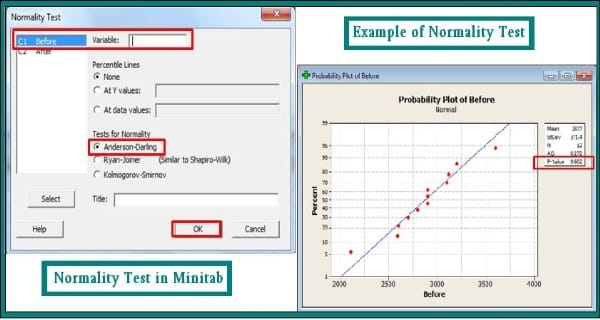Normality is one of the major concepts in statistics used for various statistical calculations. Normality Test helps one to determine whether a data is following a normal distribution or not. A normal distribution is a bell-shaped curve that is symmetric about its mean. The normal distribution is the most common statistical distribution because approximate normality arises naturally in many physical, biological, and social measurement situations. Many statistical analyses require that the data come from normally distributed populations.

One can conduct a Normality test using Minitab. Minitab has statistical tools that allow one to perform statistical calculations with ease. Let’s have a look at the steps to perform a normality test using Minitab.

Step 1: Go to File menu, click Open Project and then load the data to be analyzed.
Step 2: Go to Start menu and then move to Basic Statistics.
Step 3: Click on Normality Test and then enter the variables on the respective columns.
Step 4: Click Ok. After clicking OK, Minitab generates the probability plot in a separate window.

Example of conducting a Normality Test Taking the example of Cholesterol levels at fasting, before breakfast and after breakfast levels, let’s conduct a normality test.Go to File Menu, click Open Project and then load the file including Cholesterol levels at fasting. Go to Start menu and then move to Basic Statistics. Click on Normality Test then enter the variables on the respective columns. In this example, let us test the Column which has before, data for normality. Double click on before in the left hand side box to select it.  Now we click on Anderson-Darling and then click on OK. While there are multiple kinds of normality tests available, the Anderson Darling Test is the most reliable and commonly used test. Once we click ok, Minitab generates the probability plot in a separate window. Here we can notice that since the P value is greater than .05, we can assume the “Before” data is normal.

To know more about Normality Test, you can explore Simplilearn’s Minitab with Statistics Training. Simplilearn offers Minitab training course online with Statistics.Simplilearn